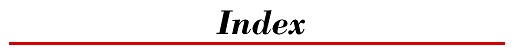start page | rating of books | rating of authors | reviews | copyrightsSymbols | A | B | C | D | E | F | G | H | I | J | K | L | M | N | O | P | R | S | T | U | V | W | Y | Z

# Symbols

& (ampersand)
& (bitwise AND) operator : Bitwise/Logical AND Operator &
&& (boolean AND) operator : Boolean AND Operator &&
* (asterisk)
in import directive : The import Directive
* (multiplication) operator : Multiplication Operator *
\ (backslash) (see Unicode characters) : Character literals
\u escapes (see Unicode characters)
! (bang)
! (unary negation) operator : Boolean Negation Operator !
!= (not-equal-to) operator : Not-Equal-To-Operator !=
[ ] (brackets)
array allocation expressions : Array Allocation Expressions
in array type declarations
Array Types
Local variable type
^ (bitwise exclusive OR) operator : Bitwise/Logical Exclusive OR Operator ^
, (comma)
Operators
The for Statement
= (equal sign)
= (assignment) operator : Assignment Operators
= = (equal-to) operator : Equal-To Operator ==
- (hyphen)
- (arithmetic subtraction) operator : Arithmetic Subtraction Operator -
- (unary minus) operator : Unary Minus Operator -
- - (decrement) operator
Field Expressions
Increment/Decrement Operators
< (left angle bracket)
< (less-than) operator : Less-Than Operator <
<= (less-than-or-equal-to) operator : Less-Than-Or-Equal-To Operator <=
<< (left shift) operator : Left Shift Operator <<
( ) (parentheses) : Parenthetical Expressions
cast operations : Casts
object allocation expressions : Object Allocation Expressions
% (remainder) operator : Remainder Operator %
+ (plus)
+ (string concatentation) operator
null
String Concatenation Operator +
+ (unary plus) operator : Unary Plus Operator +
++ (increment) operator
Field Expressions
Increment/Decrement Operators
?: (conditional) operator : Conditional Operator
> (right angle bracket)
> (greater-than) operator : Greater-Than Operator >
>= (greater-than-or-equal-to) operator : Greater-Than-Or-Equal-To Operator >=
>> (right shift) operator : Right Shift Operator >>
>>> (unsigned right shift) operator : Unsigned Right Shift Operator >>>
; (semicolon) : Method implementation
/ (slash)
/ (division) operator : Division Operator /